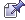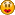###Author Topic: PHP LaTeX Rendering  (Read 20024 times)

0 Members and 1 Guest are viewing this topic.

####Sidoh

• x86
• Hero Member
••• Posts: 17668
• MHNATY ~~~~~##### PHP LaTeX Rendering
« on: October 05, 2006, 10:51:16 PM »
http://latex.sidoh.org/ui.php

Feel free to use it.  It may make your questions that much more readable!

Turn this:

\displaystyle W_{1\rightarrow2} = \int_{x_1}^{x_2}F_x\left(x\right)dx

Into this!Some other examples\displaystyle \int x^n=\frac{x^{n+1}}{n+1}\displaystyle \frac{d}{dx}x^n=nx^{n-1}

You can also pass ?render=<LaTeX> to latex.sidoh.org/ to produce an image.  Navigating to latex.sidoh.org without any parameters will redirect you to the "UI."

Notes / Tips --

• If you're having problems with a certain portion of a TeX string (ie, some character isn't showing), try encoding the URL variable in base64 and passing ?render64=<Base 64> instead (click the checkbox in the ui).
• Some tags/functionality of TeX are disabled for security reasons.
• If you're getting a broken image, that means you did something wrong.  Make sure you spelled everything correctly and that it's not too long (there's a limit to how long a LaTeX formula can be for this).
« Last Edit: October 06, 2006, 12:47:41 PM by Sidoh »

####d&q

• Hero Member
••• Posts: 1429
• I'm here.##### Re: PHP LaTeX Rendering
« Reply #1 on: October 06, 2006, 12:33:48 AM »
This will be really helpful at school, thank you!The writ of the founders must endure.

####rabbit

• x86
• Hero Member
••• Posts: 8098
• I speak for the entire clan (except Joe)##### Re: PHP LaTeX Rendering
« Reply #2 on: October 06, 2006, 08:43:51 AM »
There needs to be manual page with all the options.

####rabbit

• x86
• Hero Member
••• Posts: 8098
• I speak for the entire clan (except Joe)##### Re: PHP LaTeX Rendering
« Reply #3 on: October 06, 2006, 08:44:50 AM »
You should also tell me how to display stuff like function pi and congruence.

####Sidoh

• x86
• Hero Member
••• Posts: 17668
• MHNATY ~~~~~##### Re: PHP LaTeX Rendering
« Reply #4 on: October 06, 2006, 12:17:55 PM »
There needs to be manual page with all the options.

It's LaTeX...

You should also tell me how to display stuff like function pi and congruence.\cong\displaystyle \prod_{i=m}^{n}

####Sidoh

• x86
• Hero Member
••• Posts: 17668
• MHNATY ~~~~~##### Re: PHP LaTeX Rendering
« Reply #5 on: October 10, 2006, 05:05:58 PM »
I've been playing around with it for the last few days and you can do some pretty neat things!

Code: [Select]
$$Surface area and volume of a sphere\displaystyle \\* \indent V_{sphere}=\frac{4}{3}\pi r^{3} \\ \indent A_{sphere}=4\pi r^{2}$$\\$Volume of a cone$\displaystyle \\* \indent V_{cone}=\frac{1}{3}\pi r^{2}h$\\$ Volume of a cylinder$\displaystyle \\* \indent V_{cylinder}=\pi r^2 h$$Code: [Select] $$Trigonometric identities$\\ \indent \cos^{2}{\theta}+\sin^{2}{\theta}=1 \\ \indent 1+\tan^{2}{\theta}=\sec^{2}{\theta} \\ \indent \cot^{2}{\theta}+1=\csc^{2}{\theta}$$\\Double-angle formulas \\ \indent \sin{2\theta}=2\sin{\theta}\cos{\theta} \\ \indent \cos{2\theta}=\cos^{2}{\theta}-sin^{2}{\theta}$$\$Code: [Select]
$$\begin{center} {\bf Chain Rule} \end{center} \\\\If f(u) is differentiable at u=g(x) and g(x) is differentiable at x, then\\ \displaystyle \indent \frac{d}{dx}f(g(x))=f'(g(x))g'(x)$$####Sidoh

• x86
• Hero Member
••• Posts: 17668
• MHNATY ~~~~~##### Re: PHP LaTeX Rendering
« Reply #6 on: April 04, 2009, 03:44:46 AM »
I threw together a few changes.  I wrote a few lines of mod_rewrite so that you can access renders with a URL that doesn't look like it's dynamic.  This is useful for stupid forums that don't allow dynamically generated images to be embedded.

This is accomplished with the "short" option that appears on the UI now.

Example:( http://latex.sidoh.org/d65087fc425aa636b0666b11723b9bd5f925556f/image.gif )

You can also access the LaTeX that was used to generate the image:
http://latex.sidoh.org/d65087fc425aa636b0666b11723b9bd5f925556f/tex.txt
Code: [Select]
\displaystyle \sum_{i=0}^{\infty}\frac{1}{2^i}=2
nslay, if you read this, let me know of some of the things that you'd specifically like to see working, and I can look into it.

Note that this is separate from the plugin we're currently using for the forum, but if I can get this working nicely without too much effort, we can change that.